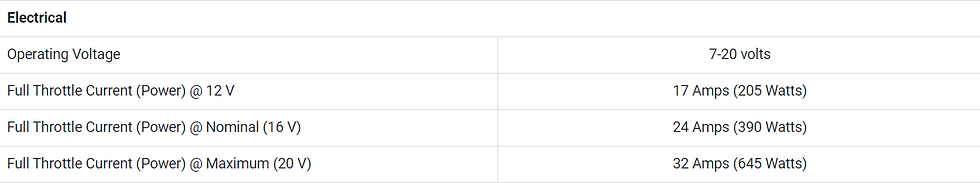top of page
• UFRJ Nautilus

# Propulsion System Control Analysis

1. Pulse Width Modulation

Pulse Width Modulation, better known as PWM (Pulse Width Modulation), is a very widespread technique in several areas of electronics, enabling the control of power and speed of components such as motors through the width of square waves, as shown in Figure 1.Figure 1 : Pulse Width Modulation Demonstration

2. Electric Current

Electric current, represented by the letter 'l', is the flow of electric charge in a given period of time, usually measured in Coulombs per second [C/s], better known today as Ampere [A]. It has a direct relationship with the acting electric potential energy (voltage) and the impedance imposed on the conductor (resistance), as shown in Figure 2.Figure 2: Ohm's Law Graph

3. Mechanical Propulsion

The mechanical propulsion of an engine for underwater vehicles, measured in kilogram-force [kgf] in the current situation, is a reaction to the movement of a propeller in the water, which pushes a column of water backwards. When this occurs, there is a reaction on the part of the spine, which pushes the propeller forward, causing the vehicle to move, as shown in Figure 3. This phenomenon can be described by Newton's Third Law. Furthermore, it is possible to convert the mechanical propulsion of an engine to Newtons by dividing the kilogram-force value by the acceleration due to gravity.Figure 3: Demonstration of Forces Acting on a Submarine

4. Thruster Model

The propeller model used by the team is the T200, manufactured by the company BlueRobotics. Figure 4 describes the electrical specifications of the thruster and Figures 5 and 6 present, respectively, the power consumption graphs as a function of the propulsion generated and the number of revolutions per minute (RPM) as a function of the PWM supplied to the ESC.Figure 4: Thruster Electrical SpecificationsFigure 5: Graph of Power as a Function of PropulsionFigure 6: RPM graph as a function of PWM

5. Current Ratio - PWM

Figure 7 shows the relationship between the electric current supplied to the thruster and the PWM supplied to the ESC responsible for its control.Figure 7: Graph of Current as a Function of PWM

6. Propulsion Ratio - PWM

Figure 8 presents the relation between the generated propulsion and the PWM supplied to the ESC responsible for the thruster control.Figure 8: PWM Propulsion Graph in Fundão

References:

Pulse-width Modulation. Wikipedia, 2023. Disponível em: https://en.wikipedia.org/wiki/ Pulse-width_modulation. Acesso em: 21/01/2023.

T200 Thruster. BlueRobotics, 2023. Disponível em: https://bluerobotics.com/store/thrusters/ t100-t200-thrusters/t200-thruster-r2-rp/. Acesso em: 21/01/2023.

CLEBSH, A. B. Propulsão Mecânica. UFRGS, 2000. Disponível em: https://ppgenfis.if. ufrgs.br/mef004/20021/Angelisa/mecanica.html. Acesso em: 21/01/2023.